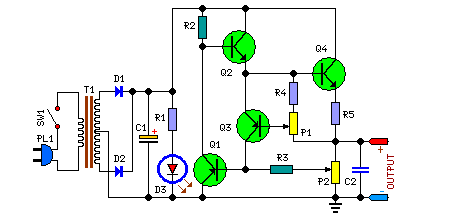# Learning Electronics

Learn to build electronic circuits

# Variable Dc Power Supply

Voltage Range: 0.7V to 24V, Current Range: 50mA to 2A

A variable dc power supply is one of the most useful tools on the electronics hobbyist's workbench. This circuit is not an absolute novelty, but it is simple, reliable, "rugged" and short-proof, featuring variable voltage up to 24V and variable current limiting up to 2A. You can adapt it to your own requirements as explained in the notes below.

Circuit Diagram :Parts:

P1 = 500R
P2 = 10K
R1 = 2.2K-1/2w
R2 = 2.2K-1/2w
R3 = 330R
R4 = 150R
R5 = 1R-5W
C1 = 35V-3300uF
D1 = 1N5402
D2 = 1N5402
D3 = 5mm Red Led
C2 = 63V-1uF
Q1 = BC182
Q2 = BD139
Q3 = BC212
Q4 = 2N3055
SW1 = SPST Mains Switch
T1 = 36VCT-Transformer

Notes:
• P1 sets the maximum output current you want to be delivered by the power supply at a given output voltage.
• P2 sets the output voltage and must be a logarithmic taper type, in order to obtain a more linear scale voltage indication.
• You can choose the Transformer on the grounds of maximum voltage and current output needed. Best choices are: 36, 40 or 48V center-tapped and 50, 75, 80 or 100VA.
• Capacitor C1 can be 2200 to 6800µF, 35 to 50V.
• Q4 must be mounted on a good heatsink in order to withstand sustained output short-circuit. In some cases the rear panel of the metal box in which you will enclose the circuit can do the job.
• The 2N3055 transistor (Q4) can be replaced with TIP3055 type.

Source: Red Free Circuit Design